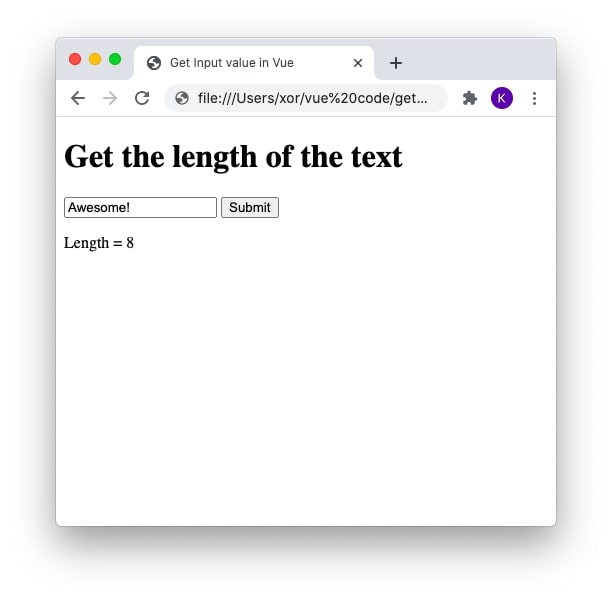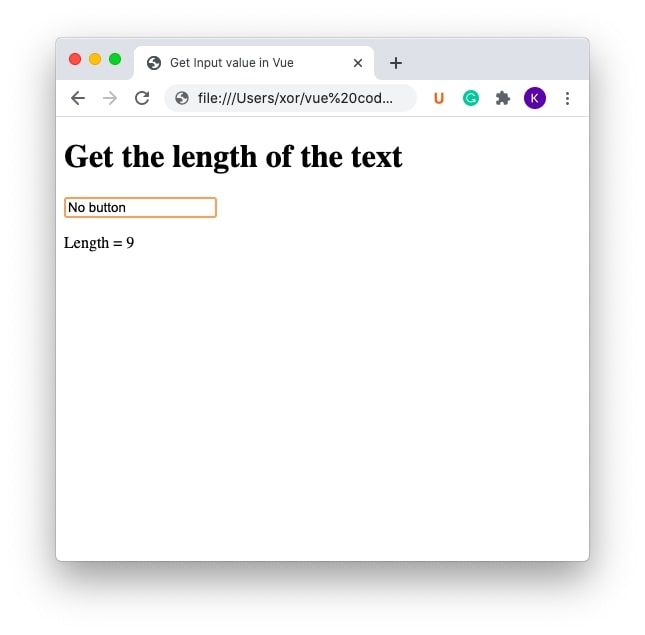If you are just getting started with Vue, one of the common questions that you will find yourself asking is how to get the value of the input field? In this article, we will look at how easy it is to obtain input value in Vue.

## Using v-model directive#

The v-model directive in Vue creates a two-way binding on the input element. All you need to do is simply declare a v-model directive for the input element and reference it to get the value.

``````<input type="text" v-model="myInput">
<p>{{ myInput }}</p>
``````

Every time the input value changes, “myInput” model will keep track of the changes.

``````<!DOCTYPE html>
<html>
<title> Get Input value in Vue</title>
<script src="https://cdn.jsdelivr.net/npm/vue/dist/vue.js"></script>
<body>
<div id="app">
<input type="text" v-model="myInput">
<p>{{ myInput }}</p>
</div>

<script>
new Vue({
el: "#app",
data: {
myInput: ""
}
})
</script>
</body>
</html>
``````

## How to pass an input value to a function in Vue#

In Vue, v-model stores the input value and there is no need to explicitly pass the value to a Vue instance method. You can directly call the v-model name in the method that needs the input value.

### Get input value on click in Vue#

Using the v-on:click or the shorthand @click we can call a function on click event. Let us look at an example to see how to get input value on click of a button. We will define an input element which takes the user input and displays the length of the input on the click of the ‘submit’ button. We will call a function “getLength” which will update the length of the text value entered.

``````<!DOCTYPE html>
<html>
<title> Get Input value in Vue</title>
<script src="https://cdn.jsdelivr.net/npm/vue/dist/vue.js"></script>
<body>
<div id="app">
<h1>Get the length of the text</h1>
<input type="text" v-model="myInput">
<button @click="getLength">Submit</button>
<p>Length = {{ length }}</p>
</div>

<script>
new Vue({
el: "#app",
data: {
myInput: "",
length: 0
},
methods: {
getLength: function () {
this.length = this.myInput.length;
}
}
})
</script>
</body>
</html>
``````In the above example, we have did not pass the value of input as parameter to method ‘getLength’.

``````getLength: function () {
this.length = this.myInput.length;
}
``````

The v-model is referenced in the method ‘getLength’ as ‘this.myInput’ which holds the current value of the input.

### Get input value on change in Vue#

Using the v-on:input or the shorthand @input we can call a function on change of input value. In our example, we can call ‘getLength’ method on the input element for every keypress without the need of button to calculate the length.

``````<!DOCTYPE html>
<html>
<title> Get Input value in Vue</title>
<script src="https://cdn.jsdelivr.net/npm/vue/dist/vue.js"></script>
<body>
<div id="app">
<h1>Get the length of the text</h1>
<input type="text" v-model="myInput" @input="getLength">
<p>Length = {{ length }}</p>
</div>

<script>
new Vue({
el: "#app",
data: {
myInput: "",
length: 0
},
methods: {
getLength: function () {
this.length = this.myInput.length;
}
}
})
</script>
</body>
</html>
``````### Get input value on enter in Vue#

The v-model directive binds the input element in such a way that it get synced with each input event. In case, you need the v-model to only sync when an event occurs, make use of the lazy modifier. This will delay the sync until the specified event is triggered.

``````<input type="text" v-model="myInput" @keyup.lazy.enter="getLength">
``````

The getLength function gets called only after ‘enter’ key is pressed.

``````<!DOCTYPE html>
<html>
<title> Get Input value in Vue</title>
<script src="https://cdn.jsdelivr.net/npm/vue/dist/vue.js"></script>
<body>
<div id="app">
<h1>Get the length of the text</h1>
<input type="text" v-model="myInput" @keyup.lazy.enter="getLength">
<p>Length = {{ length }}</p>
</div>

<script>
new Vue({
el: "#app",
data: {
myInput: "",
length: 0
},
methods: {
getLength: function () {
this.length = this.myInput.length;
}
}
})
</script>
</body>
</html>
``````

## Get input value using ref#

The v-model is the preferred way of accessing the input value in Vue. However, we can also make use ‘ref’ attribute to get the input element’s value. The ref attribute can be used on any DOM element and \$refs object is used to get that reference element’s content.

``````<!DOCTYPE html>
<html>
<title> Get Input value in Vue</title>
<script src="https://cdn.jsdelivr.net/npm/vue/dist/vue.js"></script>
<body>
<div id="app">
<h1>Get the length of the text</h1>
<input type="text" ref="myInput" @keyup="getLength">
<p>Length = {{ length }}</p>
</div>

<script>
new Vue({
el: "#app",
data: {
input: "",
length: 0
},
methods: {
getLength: function () {
this.length = this.\$refs.myInput.value.length;
}
}
})
</script>
</body>
</html>
``````